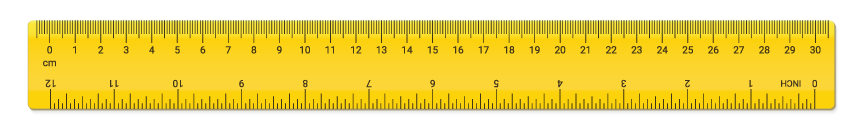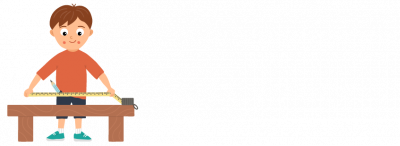How do we measure objects?

Length is how long or tall something is. It is usually measured in millimetres (mm), centimetres (cm) or metres (m).

If you take a look at a ruler you will see lots of tiny lines. The smallest lines are millimetres and the bigger lines are centimetres (cm).There are 10 mm in 1 cm.

That means:

• 10 mm = 1 cm
• 20 mm = 2 cm
• 90 mm = 9 cm
• 100 mm = 10 cm

Most rulers only measure objects up to 30 cm.

When we measure big objects we normally measure in metres (m).

We can use a tape measure to measure larger objects.There are 100 cm in 1 m.

That means:

• 100 cm = 1 m
• 200 cm = 2 m
• 900 cm = 9 m
• 1000 cm =10 m

Example 1:

Which of these measurements is the longest?

3 cm, 9 m, 20 cm, 14 m

The answer is 14 m (14 m is the same as 1400 cm).

Example 2:

Which of these measurements is the shortest?

13 mm, 24 cm, 50 mm, 8 cm

The answer is 50 mm (50 mm is the same as 5 cm).